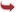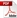International Journal of Scientific and Research Publications

#### IJSRP, Volume 6, Issue 5, May 2016 Edition [ISSN 2250-3153]Split-Deflection Method of Classical Rectangular Plate Analysis
Ibearugbulem, Owus M., Ibearugbulem, C. N, Habib Momoh and Asomugha, U.C
Abstract: This paper presents an split-deflection method of classical rectangular plate analysis. In this method, the deflection was split into x and y components of deflection. It was assumed that the deflection of the rectangular plate is the product of these two components. With this assumption, total potential energy functional was derived from principles of theory of elasticity. By direct variation of the potential energy functional, direct governing equation was obtained. Polynomial deflection and trigonometric deflection were respectively used for the x and y components of the deflection for ssss plate. This deflection components were substituted into this direct governing equation and the coefficient of the deflection was obtained to be 0.0132629qa4/D. With this coefficient, the maximum central deflection of the plate was obtained to be 0.0041446qa4/D. This was compared with the maximum central deflection of Navier’s (0.00416qa4/D) and Levi’s(0.00406qa4/D) plates. It was observed that the value from the present study makes lower bound and upper bound differences of 0.37% and 2.08% with the values from Navier and Levi respectively. This shows that this present method is reliable.
[VIEW FULL PAPER][DOWNLOAD]

Reference this Research Paper (copy & paste below code):

Ibearugbulem, Owus M., Ibearugbulem, C. N, Habib Momoh and Asomugha, U.C (2018); Split-Deflection Method of Classical Rectangular Plate Analysis; Int J Sci Res Publ 6(5) (ISSN: 2250-3153). http://www.ijsrp.org/research-paper-0516.php?rp=P535354